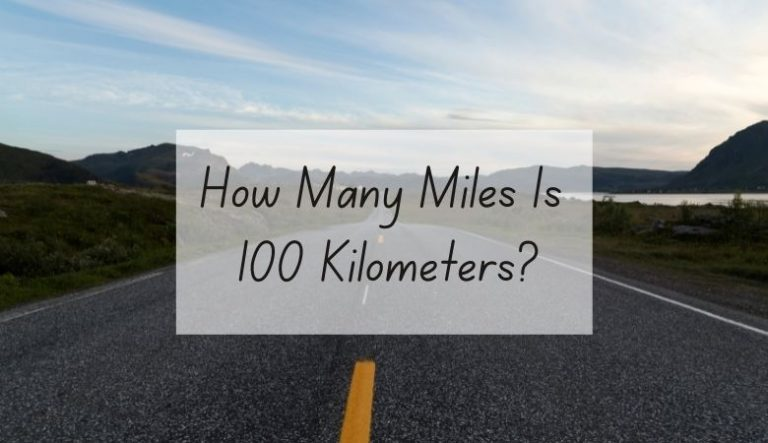Intro text, can be displayed through an additional field

## How Many Kilometers is 100 Miles?

When it comes to conversions between different units of measurement, it's important to have a clear understanding of the values involved. One common conversion that often arises is the conversion between miles and kilometers. In this article, we will explore how many kilometers are equivalent to 100 miles and provide a comprehensive guide to help you understand this conversion. So, let's dive in!

### Understanding Miles and Kilometers

Before we delve into the conversion, let's clarify what miles and kilometers represent:

#### What is a Mile?

A mile is a unit of distance commonly used in the United States, the United Kingdom, and a few other countries. It is part of the Imperial system and is equal to 5,280 feet or 1,609.34 meters.

#### What is a Kilometer?

A kilometer, on the other hand, is a unit of distance used in most countries around the world. It is part of the metric system and is equal to 1,000 meters. The metric system is widely adopted due to its simplicity and ease of conversion between units.

### The Conversion: How Many Kilometers is 100 Miles?

To convert miles to kilometers, we need to know the conversion factor between the two units. The conversion factor for miles to kilometers is 1.60934, which means that there are 1.60934 kilometers in one mile.

Now, let's calculate how many kilometers are equivalent to 100 miles:

100 miles * 1.60934 kilometers/mile = 160.934 kilometers

Therefore, 100 miles is equal to approximately 160.934 kilometers.

#### Q: How many kilometers are there in 100 miles?

A: There are approximately 160.934 kilometers in 100 miles.

#### Q: Is the conversion from miles to kilometers precise?

A: The conversion factor used to convert miles to kilometers is an approximate value. It is derived from the exact conversion factor of 1 mile = 1.609344 kilometers. However, for practical purposes, the approximation of 1.60934 is widely used and provides a close enough estimation for most everyday calculations.

#### Q: Why do some countries use miles while others use kilometers?

A: The choice between miles and kilometers as the unit of distance measurement is primarily based on historical reasons and cultural preferences. The United States and the United Kingdom, for example, continue to use miles due to their historical adoption of the Imperial system. In contrast, most other countries adopted the metric system and use kilometers as the standard unit of distance.

### Conclusion

In conclusion, 100 miles is equal to approximately 160.934 kilometers. This conversion is based on the conversion factor of 1.60934 kilometers per mile. While the conversion factor is approximate, it provides a close estimation for most practical calculations. Understanding the relationship between miles and kilometers is essential when dealing with measurements in different units. Whether you're planning a road trip or studying in a country that uses a different unit of distance, knowing how to convert between miles and kilometers is a valuable skill.

## Related video of how many kilometers is 100 miles

Ctrl
Enter
Noticed oshYwhat?
Highlight text and click Ctrl+Enter
We are in
Search and Discover » how many kilometers is 100 miles
Update Info